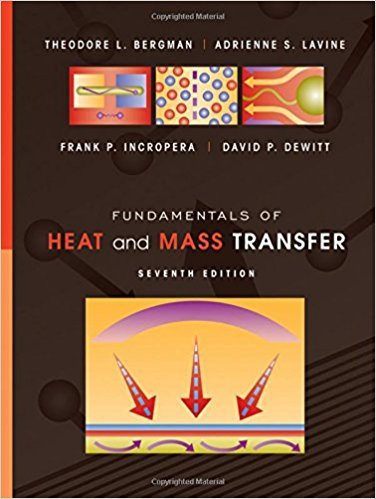×

# Consider an object of characteristic length 0.01 m and## Problem 9.3 Chapter 9

Fundamentals of Heat and Mass Transfer | 7th Edition

• 2901 Step-by-step solutions solved by professors and subject experts
• Get 24/7 help from StudySoup virtual teaching assistantsFundamentals of Heat and Mass Transfer | 7th Edition

4 5 0 296 Reviews
25
5
Problem 9.3

Consider an object of characteristic length 0.01 m and asituation for which the temperature difference is 30C.Evaluating thermophysical properties at the prescribedconditions, determine the Rayleigh number for the fol-lowing fluids: air (1 atm, 400 K), helium (1 atm, 400 K),glycerin (285 K), and water (310 K).

Step-by-Step Solution:
Step 1 of 3
Step 2 of 3

Step 3 of 3

##### ISBN: 9780470501979

Since the solution to 9.3 from 9 chapter was answered, more than 317 students have viewed the full step-by-step answer. The answer to “Consider an object of characteristic length 0.01 m and asituation for which the temperature difference is 30C.Evaluating thermophysical properties at the prescribedconditions, determine the Rayleigh number for the fol-lowing fluids: air (1 atm, 400 K), helium (1 atm, 400 K),glycerin (285 K), and water (310 K).” is broken down into a number of easy to follow steps, and 46 words. Fundamentals of Heat and Mass Transfer was written by and is associated to the ISBN: 9780470501979. The full step-by-step solution to problem: 9.3 from chapter: 9 was answered by , our top Engineering and Tech solution expert on 09/04/17, 10:17PM. This full solution covers the following key subjects: atm, prescribedconditions, glycerin, characteristic, consider. This expansive textbook survival guide covers 14 chapters, and 1569 solutions. This textbook survival guide was created for the textbook: Fundamentals of Heat and Mass Transfer, edition: 7.

Unlock Textbook Solution

Consider an object of characteristic length 0.01 m and

×
Get Full Access to Fundamentals Of Heat And Mass Transfer - 7 Edition - Chapter 9 - Problem 9.3

Get Full Access to Fundamentals Of Heat And Mass Transfer - 7 Edition - Chapter 9 - Problem 9.3